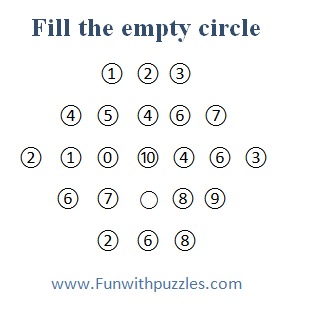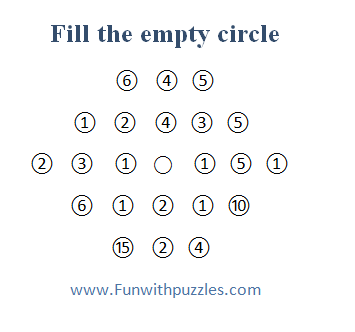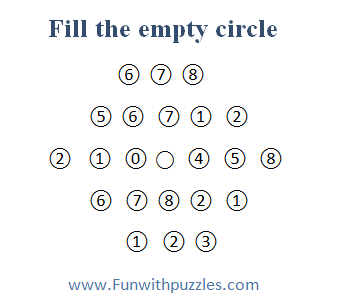## Sunday, May 17, 2020

Math Brain Teasers are very much fun to solve. Similarly, Logical Brain Teasers are very entertaining and mind refreshing. If one combines logic with fun to create Mathematical and Logical Brain Teasers then there is a blast of fun and entertainment.
Here are some challenging brain teasers which will first require you to think of a logical pattern along with Mathematical calculations to reach the correct answer. In each of the following brain teasers, one has to find out the value of the empty circle. This empty circle is surrounded by other numbers. One has to lot some of the Mathematical operations on the numbers around this empty circle to calculate the correct value of the empty circle.
Answers of these brain teasers along with a detailed explanation of each puzzle are given at the end of this post. Feel free to post your comments about these brain teasers. Do let me know if you would like to see more puzzles of this type by posting your comments in the Comments section of this post.1. Math and Logic Picture Brain Teaser2. Math and Logic Picture Brain Teaser3. Math and Logic Picture Brain Teaser4. Math and Logic Picture Brain Teaser5. Math and Logic Picture Brain Teaser

Don't forget to checkout followings Brain Teasers and Puzzles to twist your brain.

## List of Mathematical Brain Teasers and Puzzles

Answers of these Mathematical and Logical Brain Teasers are given below.
Ans 1.
4
The sum of the numbers on the right side of the middle circle -  Sum of the numbers on the left of the middle circle.

Ans 2.
4
Multiplication of all the numbers in a row  = 120

Ans 3.
0
The sum of the squares of numbers on the right side of the middle circle -  Sum of the squares of the numbers on the left of the middle circle.

Ans 4.
8
The square root of the multiplication of the numbers on the right and numbers on the left side of the middle circle

Ans 5.
10

Sum of  all the numbers on the right and left side of the middle circle / 2

1.2.1.